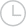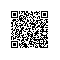# 利用RSA算法运算，如果p=11，q=13, e=103,对明文3进行加密.求d及密文-问答-阿里云开发者社区-阿里云

## 利用RSA算法运算，如果p=11，q=13, e=103,对明文3进行加密.求d及密文知与谁同2018-07-19 13:20:382576取消 提交回答

• 行者武松
2019-07-17 22:56:03
取2个质数p=11，q=13，p和q的乘积为n=p×q=143，算出φ(n)=n-p-q+1=120；再选取一个与φ(n)互质的数，例如e=7，则公开密钥=n，e=143，7.
对于这个e值，用欧几里德扩展算法可以算出其逆：d=103.因为e×d=7×103=721，满足e×d mod z =1；即721 mod 120=1成立.则秘密密钥=n，d=143，103，
设发送方需要发送机密信息（明文）m=3，发送方已经从公开媒体得到了接收方的公开密钥n，e=143，7，于是发送方算出加密后的密文c= m的e次方 mod n=42
0 0使用钉钉扫一扫加入圈子
+ 订阅